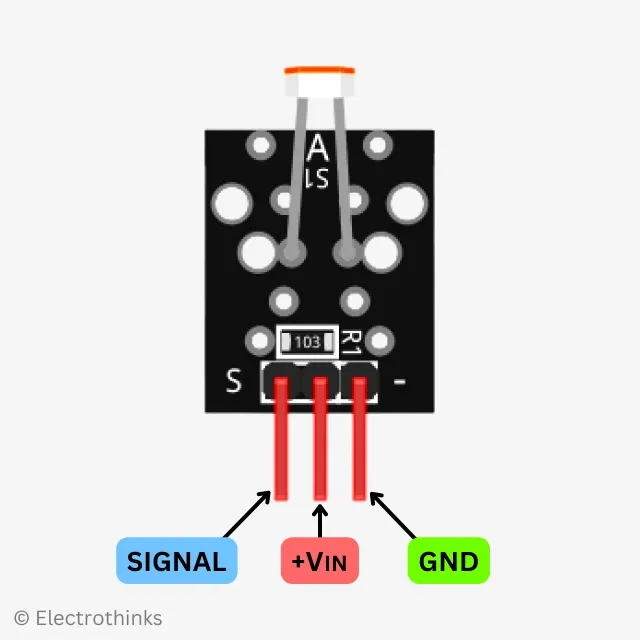# Photoresistor Module for Arduino

The Photoresistor module is a light sensor based on a photoresist, also known as a light-dependent resistor (LDR) or photocell, which is a type of resistor that changes its resistance in response to the intensity of light falling on it.

This module is used for detecting and measuring the intensity of ambient light. It provides analog output signals that can be conveniently interfaced and read by microcontrollers such as Arduino, Raspberry Pi, ESP32, and others. The data is transmitted using a single-wire analog protocol.

## Photoresistor module Specifications

The quick specifications of this sensor module is given below:

• Type: Analog
• Module: Light Sensor
• Operating Voltage: DC 3.3V to 5V
• Operating Temperature Range: -30°C to +70°C [-22°F to +158°F]
• Dark resistance: 500kΩ
• Light Sencing Responsce: 30μS
• Spectrumpeak Value: 540
• Board Dimantions (L x W x H): 30mm x 15mm x 6mm
• Weight: 2gm

## Photoresistor module PinoutThe module has 3 male header pins those are - Pin (S): Analog Signal Pin (Middle): DC 3V3 ~ 5V Pin (-): GND

## Photoresistor module Circuit diagram

Schematic of the photoresistor (light sensor) module circuit is shown below.

Components are used in the circuit - S1: 5mm LDR, and R1: 10kΩ Resistor.

The LDR is connected in a voltage divider configuration with a fixed resistor (R1). The output signal (S) voltage is taken from the junction between the LDR and the fixed resistor.

The working principle of the circuit is simple. The LDR (S1) in the module acts as a variable resistor that changes its resistance based on the intensity of light falling on it. When more light is present, the resistance decreases, and when less light is present, the resistance increases. This output signal voltage is directly proportional to the light intensity, and a programmed microcontroller can easily measure it.

## How to Interface Photoresistor module with Arduino

Connection diagram of the Photoresistor module with an Arduino is shown below.

You will need to connect the power pin (middle) and ground pin (-) of the photoresistor module to +5V (or +3V3) and GND on the Arduino, respectively. The module signal pin (S) connect to analog pin A0 on the Arduino.

Photoresistor Module Arduino
S Pin A0
middle +5V
GND

The Arduino's analog input pin is used to read the voltage at the output of the voltage divider. The analog input pin can measure voltages between 0 and 5V and converts them into a digital value.

Here's a basic Arduino sketch for the Photoresistor module, allows you to measure light intensity using the LDR and prints the corresponding light intensity level.

``````const int photoresistorPin = A0; // Arduino analog input pin connected to module OUT pin (S)

void setup() {
Serial.begin(9600); // Initialize serial communication
}

void loop() {

// Map the analog value to a light intensity range (adjust the values based on your specific needs)
int lightLevel = map(sensorValue, 0, 1023, 0, 100);

Serial.print("Light Intensity: ");
Serial.print(lightLevel);
Serial.println("%");

delay(1000); // Wait for a second
}```
```

In the Arduino code, the 'analogRead()' function to read the voltage value from the analog input pin connected to the photoresistor module. The function returns a value between 0 and 1023, representing the analog voltage value.

By mapping the analog input value to the appropriate light intensity range, you can determine the level of light falling on the photoresistor. For example, you can use the 'map()' function to map the analog input value to a specific light intensity range.

Once you upload the code to your Arduino board, open the Serial Monitor in the Arduino IDE at a baud rate of 9600. You should see the measured light intensity printed every second in the Serial Monitor.

Note: You can use the mapped light intensity value to perform actions or control other components connected to the Arduino based on the detected light level. Adjust the mapping values (0, 1023, 0, 100) in the 'map()' function according to the specific characteristics of the module and the desired light intensity range you want to work with.